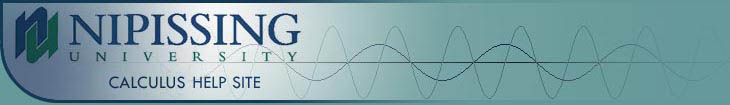TESTS
TESTS HOME

ONLINE TESTS
PRELIMINARY TESTS
GENERAL TESTS
ILRN WEBSITE

LIMITS
DERIVATIVES
RELATED RATES & OPTIMIZATION
CURVE SKETCHING
INTEGRALS
AREA & VOLUME
INVERSE FUNCTIONS

MAIN
HOME
TESTS
TUTORIALS
SAMPLE PROBLEMS
COMMON MISTAKES
STUDY TIPS
GLOSSARY
CALCULUS APPLICATIONS
MATH HUMOUR

## DERIVATIVES TEST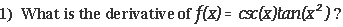a. f '(x) = -(2x)csc(x)cot(x)sec2(x2)
b. f '(x) = csc(x)[(2x)sec2(x2) -cot(x)tan(x2)]
c. f '(x) = -csc(x)[sec2(x2)-cot(x)tan(x2)]
d. f '(x) = -(2x)csc(tan(x2))cot(tan(x2))sec2(x2)a. f '(x) = -(2x)csc(x)cot(x)sec2(x2)
b. f '(x) = csc(x)[(2x)sec2(x2) -cot(x)tan(x2)]
c. f '(x) = -csc(x)[sec2(x2)-cot(x)tan(x2)]
d. f '(x) = -(2x)csc(tan(x2))cot(tan(x2))sec2(x2)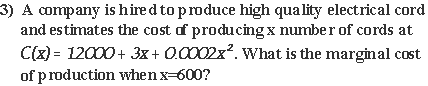a. \$3.24
b. \$13872.00
c. \$3.12
d. \$3.00a. a(0.5) = 11.0m/s2
b. a(0.5) = 12.0m/s2
c. a(0.5) = 2.66m/s2
d. a(0.5) = 24.5m/s2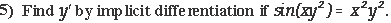a.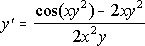b.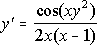c.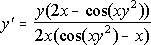d.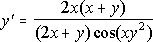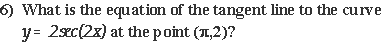a. y = 2
b. y = 0
c. y = x
d. Tangent line does not exist

If you had any trouble with this advanced test, it is strongly recommended that you review the content of the Derivatives Tutorial before attempting to take this test again. It is also recommended that you complete the General Derivatives Test on the iLrn website and the questions from the Derivatives Sample Problems page. The links for all of these resources can be found below.

Derivatives Tutorial
Derivatives Problems
General Derivatives Test on iLrn

|Top of Page |

COURSE HOMEPAGES
MATH 1036
MATH 1037

FACULTY HOMEPAGES
Alex Karassev
Ted Chase